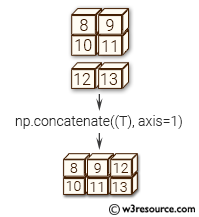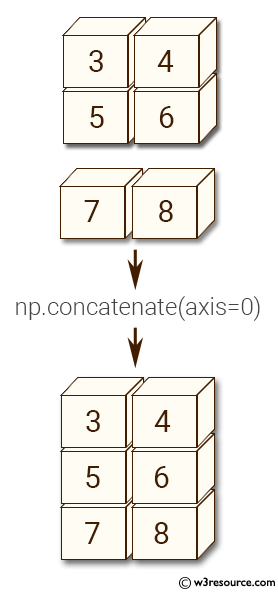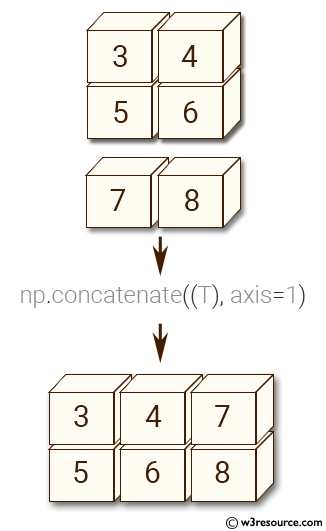# NumPy Array manipulation: concatenate() function

## numpy.concatenate() function

The concatenate() function returns an ndarray of the provided type that satisfies requirements.

Syntax:

`numpy.concatenate((a1, a2, ...), axis=0, out=None)`Version: 1.15.0

Parameter:

Name Description Required /
Optional
a1,a2 The arrays must have the same shape, except in the dimension corresponding to axis (the first, by default). Required
axis The axis along which the arrays will be joined. If axis is None, arrays are flattened before use. Default is 0. Optional
out If provided, the destination to place the result. The shape must be correct, matching that of what concatenate would have returned if no out argument were specified. Optional

Return value:

res : ndarray - The concatenated array.

Example-1: numpy.concatenate()

``````>>> import numpy as np
>>> x = np.array([[3, 4], [5, 6]])
>>> y = np.array([[7, 8]])
>>> np.concatenate((x,y), axis=0)
array([[3, 4],
[5, 6],
[7, 8]])
``````

Pictorial Presentation:Example-2: numpy.concatenate()

``````>>> import numpy as np
>>> x = np.array([[3, 4], [5, 6]])
>>> y = np.array([[7, 8]])
>>> np.concatenate((x, y.T), axis=1)
array([[3, 4, 7],
[5, 6, 8]])
``````

Pictorial Presentation:Example-3: numpy.concatenate()

``````>>> import numpy as w3r
>>> x = w3r.array([[3, 4], [5, 6]])
>>> y = w3r.array([[7, 8]])
>>> w3r.concatenate((x, y), axis=None)
array([3, 4, 5, 6, 7, 8])
``````

Example-4: numpy.concatenate()

``````>>> import numpy as w3r
>>> x = w3r.ma.arange(5)
>>> y = w3r.arange(3, 7)
>>> x
masked_array(data = [0 -- 2 3 4],
mask = [False  True False False False],
fill_value = 999999)

>>> y
array([3, 4, 5, 6])
>>> w3r.concatenate([x, y])
masked_array(data = [0 1 2 3 4 3 4 5 6],
fill_value = 999999)

>>> w3r.ma.concatenate([x, y])
masked_array(data = [0 -- 2 3 4 3 4 5 6],
mask = [False  True False False False False False False False],
fill_value = 999999)
``````

Python - NumPy Code Editor:

Previous: require()
Next: stack()

﻿